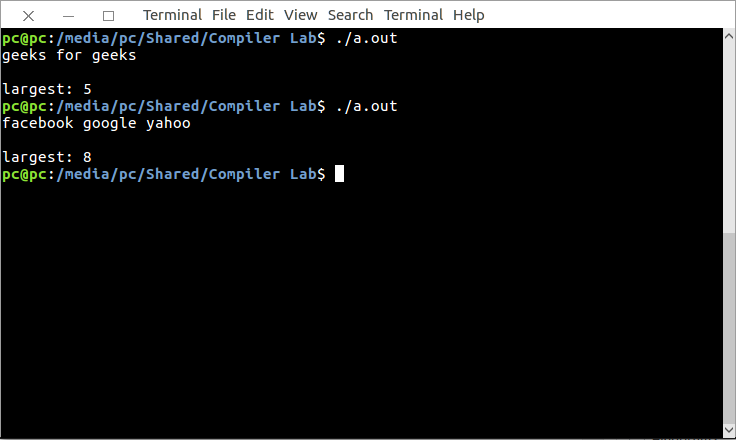Related Articles

# Lex program to find the length of the longest word

• Difficulty Level : Basic
• Last Updated : 30 Apr, 2019

Lex is a computer program that generates lexical analyzers. Lex reads an input stream specifying the lexical analyzer and outputs source code implementing the lexer in the C programming language.

The commands for executing the lex program are:

```lex abc.l (abc is the file name)
cc lex.yy.c -efl
./a.out ```

Let’s see lex program to check valid email.

Examples:

```Input:  geeks for geeks
Output:  5

Output:  8 ```

Below is the implementation:

 `/*lex code to find the length of the longest word*/`` ` `% {``  ``int` `counter = 0; %``}`` ` `%``% [a - zA - Z] + {``  ``if` `(yyleng > counter) {``    ``counter = yyleng;``  ``}``} %``%`` ` `main() {``  ``yylex();``  ``printf``(``"largest: %d"``, counter);``  ``printf``(``"\n"``);``}`

Output:My Personal Notes arrow_drop_up